### Average K.E, per molecule of a gas: kinetic interpretation of temperature.

• Consider one mole of a gas. Let P, V, T and M be the pressure, volume, temperature and molecular mass of the gas respectively.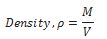• Acc. To Kinetic theory, the pressure exerted by the gas is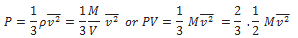but is the average Kinetic Energy E of one mole of the gas.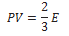• The ideal gas equation for one mole of a gas is PV =RT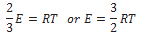The above eq. gives the mean K.E of one mole of the gas.

• If N is the Avogadro’s number, then the mean K.E per molecule is given by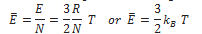Where kB = R/N is the gas constant per molecule and is called Boltzmann’s constant.

• Thus the mean kinetic energy per molecule is proportional to the absolute temperature of the gas. It is independent of the pressure, volume and the nature of the ideal gas. Clearly,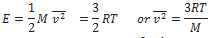• The square root of is known as root mean square velocity and is given by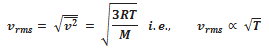• At T = 0, vrms = 0. So, we can define absolute zero as that temperature at which all molecular motion stops.